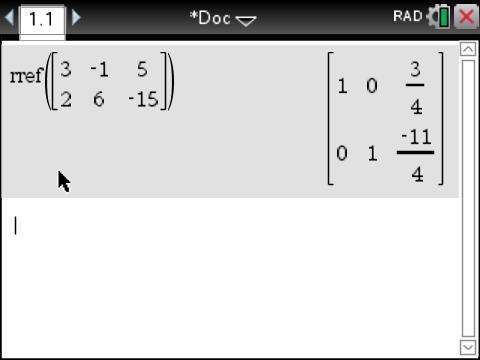# Activities

•• ##### Subject Area

• Standard: VCE: Further Mathematics: Further Mathematics

• ##### AuthorAust Senior

90 Minutes

• ##### Device
• TI-Nspire™ CAS
• TI-Nspire™ Navigator™
• ##### Software

TI-Nspire™ CAS
TI-Nspire™ CAS Navigator™ NC System

4.2

## Solving with matrices#### Activity Overview

Students use Row-Reduction in matrices to solve simultaneous equations. Simultaneous equations with no solutions and infinitely many solutions are explored using matrices and graphs.

#### Objectives

• Introduction to use of matrices to solve systems of linear equations
• Use of the ‘rref’ function as a useful tool for determining the nature of any solutions

#### Vocabulary

matrix representation, ‘rref’ function, solving systems of equations